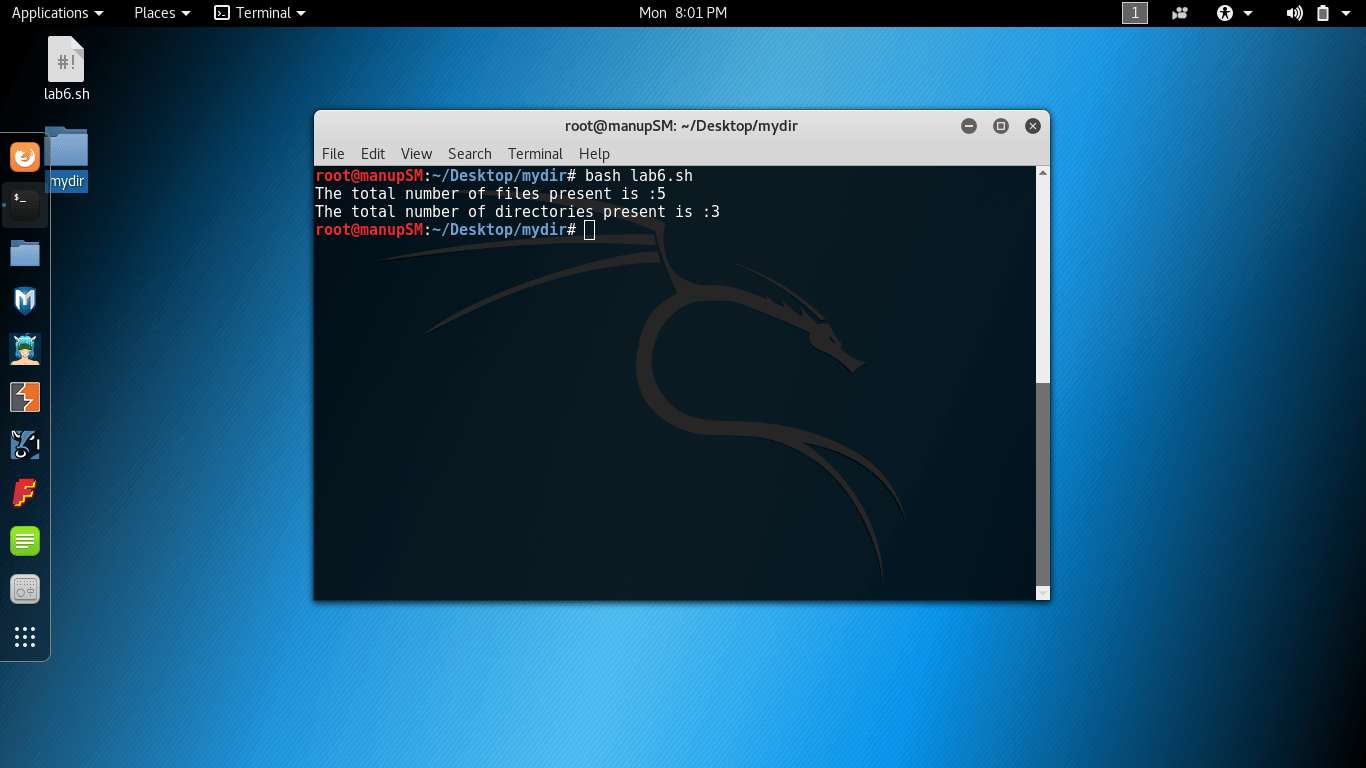# Some Shell Scripts

This post covers some of the shell scripts to:

1. Find roots of the quadratic equation

2. To count the number of files and directories in directory

3. Store the information of a student such as student name, department, year and semester in an array and display all of the information store in an array.

4. Display grade of student for marks of 5 subjects given as input

## Programs

1. Find roots of the quadratic equation

```#!bin/sh
echo -e "Enter the value of a:\c"
echo -e "Enter the value of b:\c"
echo -e "Enter the value of c:\c"
echo "The two roots of the quadratic equations are "
echo "(-\$b+sqrt(\$b^2-4*\$a*\$c))/2*\$a" | bc
echo "(-\$b-sqrt(\$b^2-4*\$a*\$c))/2*\$a" | bc
```

1. To count the number of files and directories in directory

```#!bin/sh
#Program code to compute the total number of file and folder
echo -e "The total number of files present is :\c"
ls -l | grep "^-" | wc -l
echo -e "The total number of directories present is :\c"
ls -l | grep "^d" | wc -l
```

1. Store the information of a student such as student name, department, year and semester in an array and display all of the information store in an array.

```#!bin/sh
#Program to store student info in array and then display it.
echo -e "Enter the name:\c"
echo -e "Enter the department name:\c"
echo -e "Enter the year of join:\c"
echo -e "Enter the current semester:\c"
student_info=("\$name" "\$department" "\$year" "\$semester")
echo \${student_info[@]}
```

1. Display grade of student for marks of 5 subjects given as input

```#!bin/sh
#Grading a student based on his 5 subject marks:
echo -e "Enter sub1 mark:\c"
echo -e "Enter sub2 mark:\c"
echo -e "Enter sub3 mark:\c"
echo -e "Enter sub4 mark:\c"
echo -e "Enter sub5 mark:\c"
sum=\$((\$sub1+\$sub2+\$sub3+\$sub4+\$sub5))
avg=\$(echo "\$sum/5" | bc)
if [ \$avg -ge 80 ]
then
echo Excellent. You have scored \$avg%
elif [ \$avg -ge 70 ]
then
echo Very Good. You have scored \$avg%
elif [ \$avg -ge 60 ]
then
echo Good. You have scored \$avg%
elif [ \$avg -ge 50 ]
then
echo Satisfactory. You have scored \$avg%
elif [ \$avg -ge 40 ]
then
echo Unsatisfactory. You have scored \$avg%
else
echo Failed. You have scored \$avg%
fi```